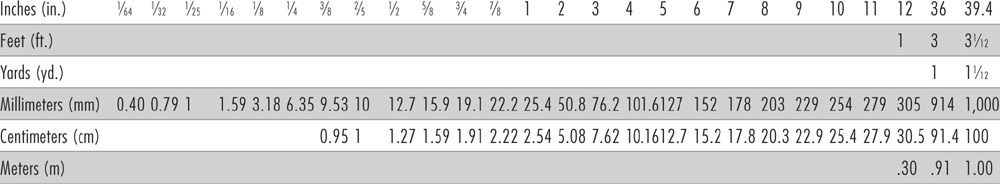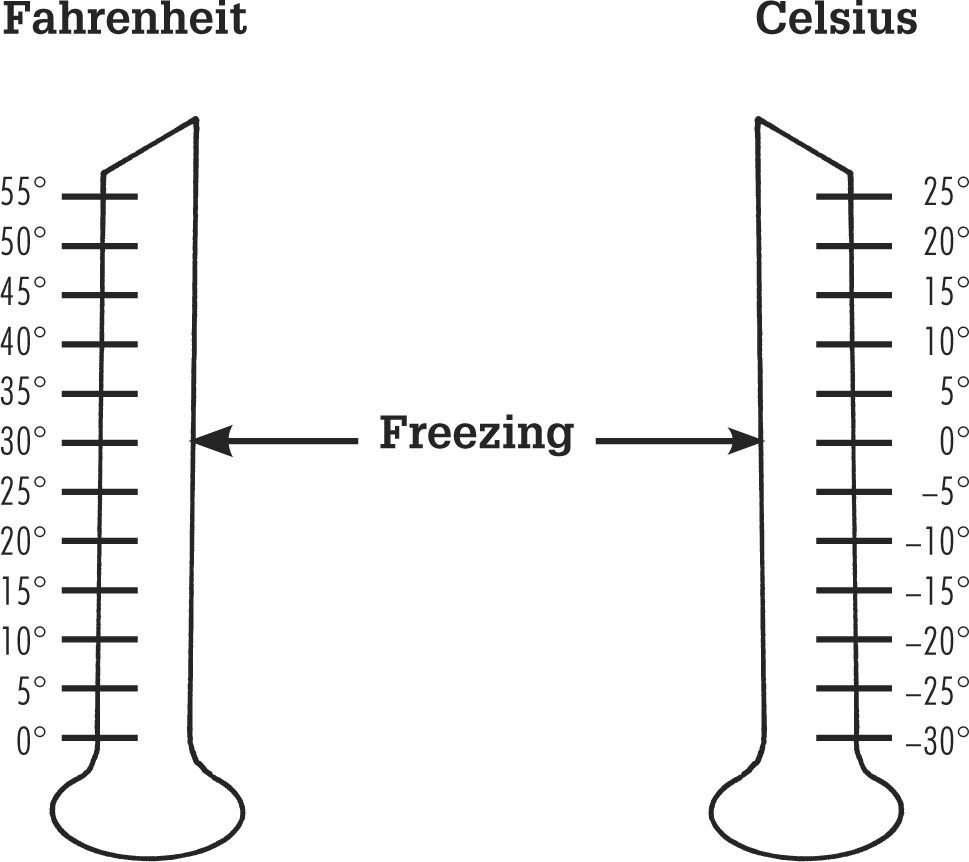﻿ ﻿Conversions - The Complete Guide to Wiring - Black & Decker, Cool Springs Press

# The Complete Guide to Wiring, Updated 6th Edition: Current with 2014-2017 Electrical Codes - Black & Decker, Cool Springs Press (2014)

### Conversions

Metric EquivalentConverting Measurements

 TO CONVERT: TO: MULTIPLY BY: Inches Millimeters 25.4 Inches Centimeters 2.54 Feet Meters 0.305 Yards Meters 0.914 Miles Kilometers 1.609 Square inches Square centimeters 6.45 Square feet Square meters 0.093 Square yards Square meters 0.836 Cubic inches Cubic centimeters 16.4 Cubic feet Cubic meters 0.0283 Cubic yards Cubic meters 0.765 Pints (U.S.) Liters 0.473 (lmp. 0.568) Quarts (U.S.) Liters 0.946 (lmp. 1.136) Gallons (U.S.) Liters 3.785 (lmp. 4.546) Ounces Grams 28.4 Pounds Kilograms 0.454 Tons Metric tons 0.907 Millimeters Inches 0.039 Centimeters Inches 0.394 Meters Feet 3.28 Meters Yards 1.09 Kilometers Miles 0.621 Square centimeters Square inches 0.155 Square meters Square feet 10.8 Square meters Square yards 1.2 Cubic centimeters Cubic inches 0.061 Cubic meters Cubic feet 35.3 Cubic meters Cubic yards 1.31 Liters Pints (U.S.) 2.114 (lmp. 1.76) Liters Quarts (U.S.) 1.057 (lmp. 0.88) Liters Gallons (U.S.) 0.264 (lmp. 0.22) Grams Ounces 0.035 Kilograms Pounds 2.2 Metric tons Tons 1.1

Converting Temperatures

Convert degrees Fahrenheit (F) to degrees Celsius (C) by following this simple formula: Subtract 32 from the Fahrenheit temperature reading. Then mulitply that number by 5/9. For example, 77°F - 32 = 45. 45 × 5/9 = 25°C.

To convert degrees Celsius to degrees Fahrenheit, multiply the Celsius temperature reading by 9/5, then add 32. For example, 25°C × 9/5 = 45. 45 + 32 = 77°F.﻿